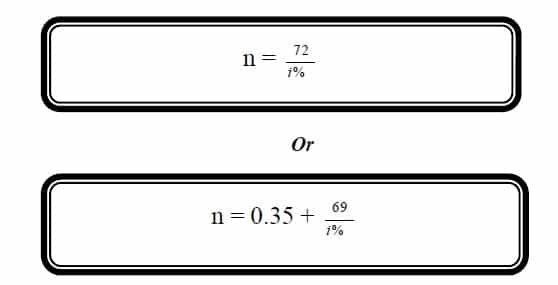Select Page

Rule of 72

Investors commonly ask the question that how long it would take to double the amount at a given rate of interest. To answer we use the rule of 72 or rule of 69.Example 1:

To estimate how long it will take Rs. 15,000 to grow to Rs. 30,000 at an annual growth rate of 10%?